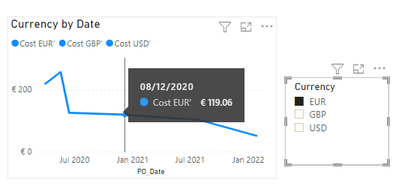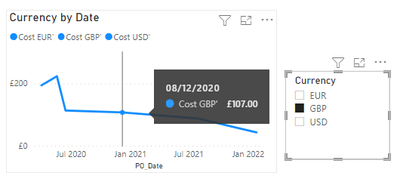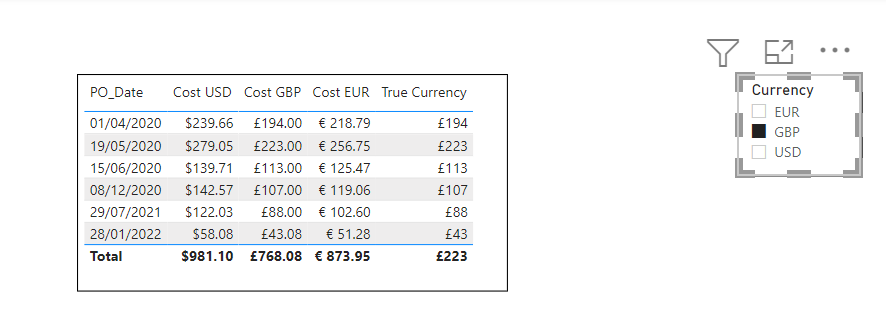cancel
Showing results for
Did you mean:Frequent Visitor

## Measure Not Correct Currency

Have a table called Cost_Data and it has costs in three different currencies USD, GBP, and EUR.  Another table called Currency_Convert with Columns Currency, Symbol, Format.  I am trying to have a slicer that you can select USD, GB, or EUR and the cost will update.  What I have works but it is not pulling the right symbol from the data.  I tried to use the formatting from my Currency_convert table but that changes it to text and it has no numerical value to use elsewhere.

My slicer is showng EUR and the number is correct for True Currency Column but the symbol is wrong.1 ACCEPTED SOLUTIONCommunity Support

The reason why the line chart is not displayed after using the new measure is that the data obtained in the FORMAT format is essentially a text string. Even if the data format is changed to number or currency, it cannot be sorted by size, but only in alphabetical order.But the Values in the line chart should be of number data type, so it can't display anything.

If you want the symbol displaied correctly in the line chart, you should have three measures, set the corresponding currency symbol for each measure, here's my solution.

``````Cost EUR' =
IF (
SELECTEDVALUE ( Currency_Convert[Currency] ) = "EUR",
MAX ( 'Cost_Data'[Cost EUR] ),
BLANK ()
)
``````
``````Cost GBP' =
IF (
SELECTEDVALUE ( Currency_Convert[Currency] ) = "GBP",
MAX ( 'Cost_Data'[Cost GBP] ),
BLANK ()
)
``````
``````Cost USD' =
IF (
SELECTEDVALUE ( Currency_Convert[Currency] ) = "USD",
MAX ( 'Cost_Data'[Cost USD] ),
BLANK ()
)
``````

It get the correct result for each currency.I attach my sample below for reference.

Best Regards,
Community Support Team _ kalyj

If this post helps, then please consider Accept it as the solution to help the other members find it more quickly.

4 REPLIES 4Community Support

The reason why the line chart is not displayed after using the new measure is that the data obtained in the FORMAT format is essentially a text string. Even if the data format is changed to number or currency, it cannot be sorted by size, but only in alphabetical order.But the Values in the line chart should be of number data type, so it can't display anything.

If you want the symbol displaied correctly in the line chart, you should have three measures, set the corresponding currency symbol for each measure, here's my solution.

``````Cost EUR' =
IF (
SELECTEDVALUE ( Currency_Convert[Currency] ) = "EUR",
MAX ( 'Cost_Data'[Cost EUR] ),
BLANK ()
)
``````
``````Cost GBP' =
IF (
SELECTEDVALUE ( Currency_Convert[Currency] ) = "GBP",
MAX ( 'Cost_Data'[Cost GBP] ),
BLANK ()
)
``````
``````Cost USD' =
IF (
SELECTEDVALUE ( Currency_Convert[Currency] ) = "USD",
MAX ( 'Cost_Data'[Cost USD] ),
BLANK ()
)
``````

It get the correct result for each currency.I attach my sample below for reference.

Best Regards,
Community Support Team _ kalyj

If this post helps, then please consider Accept it as the solution to help the other members find it more quickly.Super User

This seems to be a somewhat known issue. Since the format function translate the number to text, it works well in tables but not in graphs... I googled a bit and found this:

Solved: DAX to dynamically change the currency symbol faci... - Microsoft Power BI Community

So, my suggestion is to have two measures, one for the tables with the correct currency sign. And another one for graphs. that one still shows the correct exchange rate conversion, but it misses out on the currency symbol....Here the measures (I fixed the total values also 🙂)

```True Currency Number =
Var USD_Cost = SUM(Cost_Data[Cost USD])
Var GBP_Cost = SUM(Cost_Data[Cost GBP])
Var EUR_Cost = SUM(Cost_Data[Cost EUR])
Var Currency_ID = Cost_Data[Currency Selected]

RETURN
SWITCH(
TRUE(),
Currency_ID = "USD" , USD_Cost,
Currency_ID = "GBP" , GBP_Cost,
Currency_ID = "EUR" , EUR_Cost,
0
)```
```True Currency Text =
Var Currency_ID = Cost_Data[Currency Selected]
RETURN
SWITCH(
TRUE(),
Currency_ID = "USD" , FORMAT(Cost_Data[True Currency Number],"\$#.00"),
Currency_ID = "GBP" , FORMAT(Cost_Data[True Currency Number],"£#.00"),
Currency_ID = "EUR" , FORMAT(Cost_Data[True Currency Number],"€#.00"),
0
)```

I additionally changed the type of the measures to Currency for [True Currency Text] and to Decimal for [True Currency Number].

Proud to be a Super User!Super User

Here a solution:And here your updated DAX measure:

```True Currency =
Var USD_Cost = Max(Cost_Data[Cost USD])
Var GBP_Cost = Max(Cost_Data[Cost GBP])
Var EUR_Cost = Max(Cost_Data[Cost EUR])
Var Currency_ID = Cost_Data[Currency Selected]

Return
SWITCH(
TRUE(),
Currency_ID = "USD" , FORMAT(USD_Cost,"\$#,##0"),
Currency_ID = "GBP" , FORMAT(GBP_Cost,"£#,##0"),
Currency_ID = "EUR" , FORMAT(EUR_Cost,"€#,##0"),
0)```

Does this solve your issue? 🙂

[EDIT]

If you'd like to show two decimals, then you can use this code here:

```True Currency =
Var USD_Cost = Max(Cost_Data[Cost USD])
Var GBP_Cost = Max(Cost_Data[Cost GBP])
Var EUR_Cost = Max(Cost_Data[Cost EUR])
Var Currency_ID = Cost_Data[Currency Selected]

Return
SWITCH(
TRUE(),
Currency_ID = "USD" , FORMAT(USD_Cost,"\$#.00"),
Currency_ID = "GBP" , FORMAT(GBP_Cost,"£#.00"),
Currency_ID = "EUR" , FORMAT(EUR_Cost,"€#.00"),
0)```

Proud to be a Super User!Frequent Visitor

Thanks for the suggestion, I actually tried this and it does work for the table but it doesn't work in the chart.  I still don't understand why the chart doesn't work.   Also, in the table the total for that column is wrong also.Announcements#### Exclusive opportunity for Women!

Join us for a free, hands-on Microsoft workshop led by women trainers for women where you will learn how to build a Dashboard in a Day!#### Power Platform Conference-Power BI and Fabric Sessions

Join us Oct 1 - 6 in Las Vegas for the Microsoft Power Platform Conference.Top Solution Authors
Top Kudoed Authors
Users online (8,451)